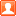##用户名 Email 自动登录 找回密码 密码 注册
 搜索 热搜: 活动 交友 discuz

# 令 an={√(n^2+1)}，其中 {x} 表示 x 的小数部分，求 lim(n→∞)nan发表于 2020-11-20 09:15 | 显示全部楼层 |阅读模式

### 本帖子中包含更多资源

x发表于 2020-11-20 10:42 | 显示全部楼层
 $a_n =\sqrt{n^2+1}-\lfloor\sqrt{n^2+1}\rfloor=\sqrt{n^2+1}-n=\large\frac{1}{n+\sqrt{n^2+1}}$ $\displaystyle\lim_{n\to\infty}na_n=\lim_{n\to\infty}{\small\frac{n}{n+\sqrt{n^2+1}}}=\small\frac{1}{2}$发表于 2020-11-20 13:46 | 显示全部楼层
 楼上 elim 的解答很好！已收藏。楼主| 发表于 2020-11-20 17:18 | 显示全部楼层
 elim老师这种写法不太大众化，可以这样：

### 本帖子中包含更多资源

x

 您需要登录后才可以回帖 登录 | 注册 本版积分规则 回帖并转播 回帖后跳转到最后一页

GMT+8, 2020-12-2 19:44 , Processed in 0.075196 second(s), 16 queries .